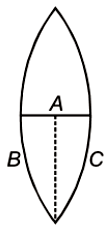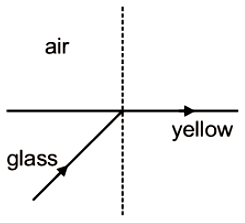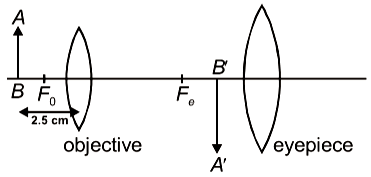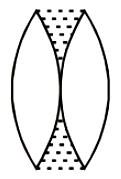The minimum distance between a real object and screen such that a convex lens of focal length 20 cm can produce a real image on the screen is.

1.  20 cm

2.  40 cm

3.  80 cm

4.  60 cm

Concept Questions :-

Lenses
High Yielding Test Series + Question Bank - NEET 2020

Difficulty Level:

A concave lens of focal length 25 cm produces image $\frac{1}{10}th$ the size of the object. The distance of the object from the lens is

(1) 225 cm

(2) 250 cm

(3) 150 cm

(4) 175 cm

Concept Questions :-

Lenses
High Yielding Test Series + Question Bank - NEET 2020

Difficulty Level:

Assume that light of wavelength 7000 Angstrom is coming from a star. The limit of resolution (in radian) of a telescope whose objective has a diameter of 244 cm, will be

1.

2.

3.

4.

Concept Questions :-

Resolution of Optical devices
High Yielding Test Series + Question Bank - NEET 2020

Difficulty Level:

Which of the following is not due to the total internal reflection of light

1.  Brilliance of diamond

2.  Mirage on hot summer days

3.  Working of optional fiber

4.  formation of rainbow

Concept Questions :-

Total internal reflection
High Yielding Test Series + Question Bank - NEET 2020

Difficulty Level:

A ray of light incident on an equilateral prism at grazing incidence emerges from the prism at grazing emergence. Refractive index of the prism is

(1) 1.5

(2) 1.8

(3) 1.33

(4) 2

Concept Questions :-

Total internal reflection
High Yielding Test Series + Question Bank - NEET 2020

Difficulty Level:

Absolute refractive indices of glass and water are 3/2 and 4/3. The ratio of velocity of light in glass and water will be

1.  4:3

2.  8:7

3.  8:9

4.  3:4

Concept Questions :-

Refraction at plane surface
High Yielding Test Series + Question Bank - NEET 2020

Difficulty Level:

A thin equiconvex lens of power P is cut into three parts A, B, and C as shown in the figure. If  are powers of the three parts respectively, then1.

2.

3.

4.

Concept Questions :-

Lenses
High Yielding Test Series + Question Bank - NEET 2020

Difficulty Level:

A light beam containing red, yellow and blue color, falls on the interface of glass air as shown. If the yellow light is just totally internally reflected, then emergent ray in air contains-1.  Both red and blue color

2.  Only red color

3.  Only blue color

4.  No color

Concept Questions :-

Total internal reflection
High Yielding Test Series + Question Bank - NEET 2020

Difficulty Level:

Focal lengths of objective and eyepiece of a compound microscope are 2 cm and 6.25 cm respectively. An object AB is placed at a distance of 2.5 cm from the objective which forms the image B'A' as shown in the figure. Maximum magnifying power in this case is :1.  10

2.  20

3.  5

4.  25

Concept Questions :-

Simple and compound microscope
High Yielding Test Series + Question Bank - NEET 2020

Difficulty Level:

Two identical equiconvex thin lenses each of focal lengths 20 cm, made of material of refractive index 1.5 are placed coaxially in contact as shown. Now, the space between them is filled with a liquid of refractive index 1.5. The equivalent power of this arrangement will be:1.  + 5D

2.  Zero

3.  + 2.5D

4.  + 0.5D

Concept Questions :-

Lensmakers' formula